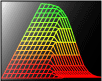The Predictive Suite Integrates Statistical and Graphical Data Analysis with State-of-the-Art Predictive Modeling

###Key Features

• Automated variable selection identifies key variables & variable interactions

• Insightful visualization of data and model dynamics

• Execution of batch commands

• SQL queries and dataset browsing

• Pre & post processing for creating variables, constraining outputs, etc.

• Models easily deployed via ActiveX (i.e., OCX) controls or DLLs

• Powerful modeling algorithms including: regression, neural networks, self-organizing maps, dynamic clustering, decision trees, fuzzy logic, genetic algorithms

See the Predictive Suite demo page here.

Predictive Dynamix Model Types

• NNDynamix -- Neural network models
• CMDynamix -- Self-organizing maps & dynamic clustering models
• FPDynamix -- Fuzzy & continuous logic models
• RGDynamix -- Regression models
• DTDynamix -- Decision tree models

See the Predictive Technology page here.

Data & Model Analysis Functionality

 Graphical Analysis Statistical Analysis Model Analysis Scatter Plots (1D, 2D, & 3D) Cross-tab Tool Variable Sensitivity Analysis Bar Charts (2D & 3D) Variable Cross Correlations Interactive "What-if" Analysis 3D Surface Plots Optimal Sampling & Data Reduction Graphical Decision Surface Line Charts ROC / Lift Charts DB Execution & Accuracy Stats